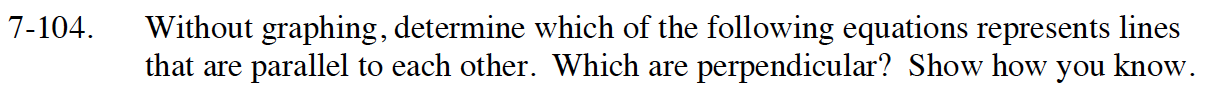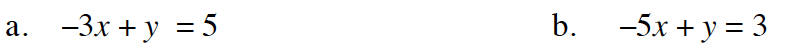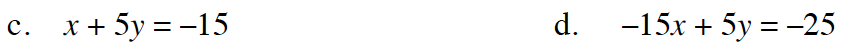Home > CC4 > Chapter 7 > Lesson 7.2.2 > Problem7-104

7-104.
1. Without graphing, determine which of the following equations represents lines that are parallel to each other. Which are perpendicular? Show how you know. Homework Help ✎

2.  a. –3x + y = 5 b. –5x + y = 3 c. x + 5y = –15 d. –15x + 5y = –25Put each equation into slope-intercept form
(y = mx + b, where m is the slope and b is the y-intercept). That means to solve for y.

Compare the slopes. If they are equal then they are parallel.
If they are the negative reciprocal of each other then they are perpendicular,